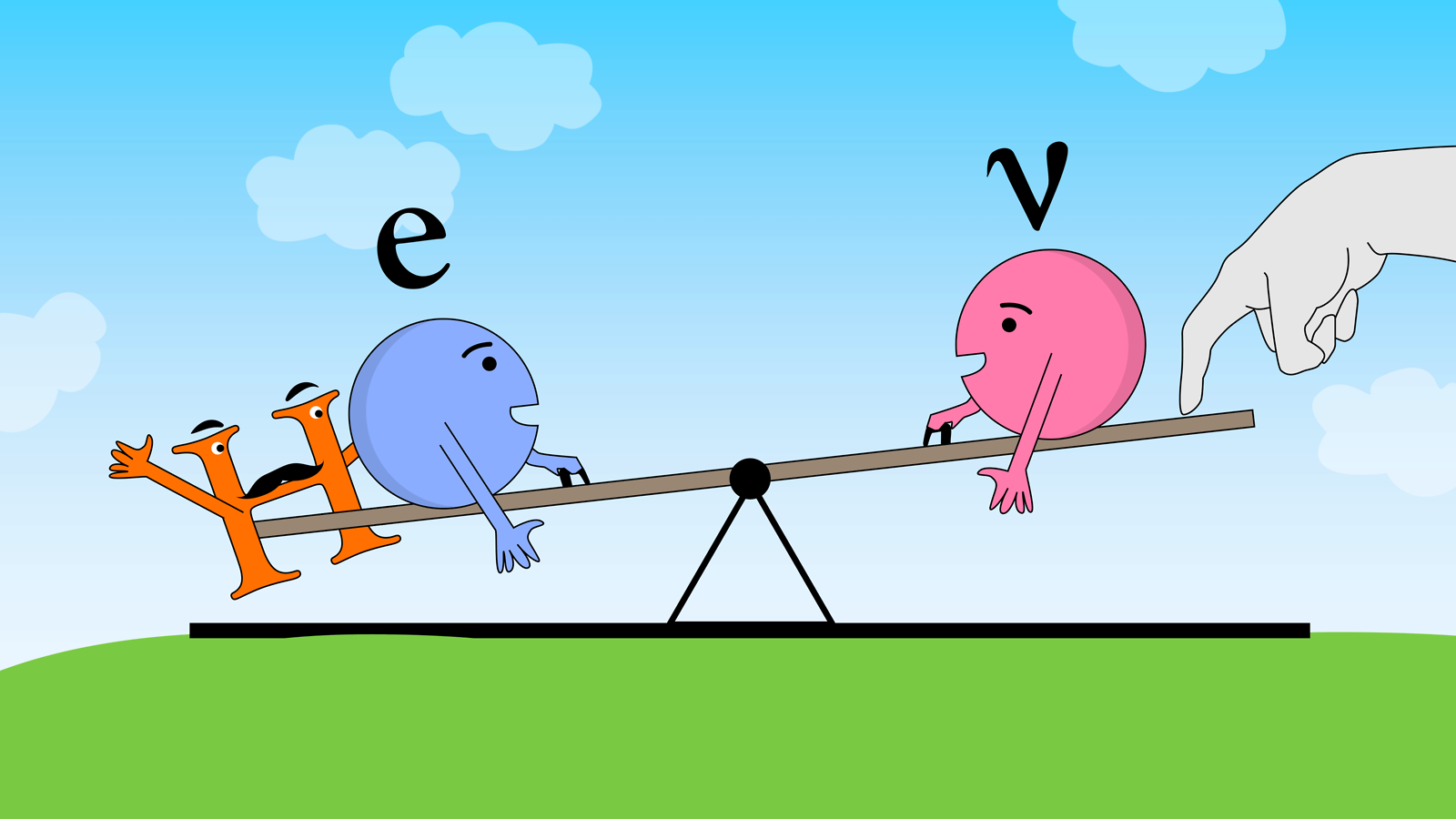A joint Fermilab/SLAC publicationIllustration by Sandbox Studio, Chicago

# Neutrinoless double beta decay

08/27/13

The observation of neutrinoless double beta decay would suggest that, by itself, the Standard Model Higgs cannot give mass to neutrinos.

Last year, physicists discovered the long-sought Higgs boson, bringing us closer to understanding why particles such as electrons have mass. Neutrinos, on the other hand, are much, much lighter than electrons and other similar particles. Could this be a sign that the story of neutrino mass is not just about the Higgs?

We can explore this possibility with a process known as double beta decay. In “normal” double beta decay, two neutrons inside an atomic nucleus transform into two protons while ejecting two neutrinos and two electrons. A dozen different nuclei have been found to undergo this very rare type of decay.

Now suppose that neutrinos, in addition to being electrically neutral, are in fact exactly neutral in all possible ways—so much so that a neutrino and an anti-neutrino are utterly identical.

If this is true, then neutrinos should fuel a “neutrinoless” type of double beta decay. In this process, the experimenter would see only the emission of two electrons, because the neutrinos would have been absorbed by the same nucleus from which they came. It’s these “missing” neutrinos that provide the name for the neutrinoless decay.

Neutrinoless double beta decay is intriguing because, by itself, the Higgs described by the Standard Model cannot give mass to this type of completely neutral neutrino.

There are, however, many other—as-yet-unproven—theories for how such neutrinos could get a small mass.  And since we know that neutrinos in nature are almost (but not quite) massless, the next step to figuring out if any of these ideas are on the right track is to demonstrate or disprove their completely neutral nature. This can best be done by observing or ruling out the neutrinoless decay.

Like what you see? Sign up for a free subscription to symmetry!